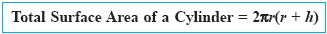Name: ___________________Date:___________________

 Email us to get an instant 20% discount on highly effective K-12 Math & English kwizNET Programs!

### Geometry6.18 Total Surface Area of a Cylinder

 Total Surface Area of a cylinder = Lateral surface area + Area of two circles (top and bottom circles)Total surface area = 2*pi*r2 + 2*pi*r*h = 2 * pi * r * (r+h).Example: The ratio of the radius and height of a cylinder is 5:3. The radius is 25cm. Find its total surface area. Solution: Given that r = 25cm. Height (h) = 3/5 * 25 = 15cm. Total surface area = 2 * pi * r * (h+r). = 2 * 22/7 * 25(25+15) = 1100/7 * 40 = 6285.71 Sq. cm. Directions: Solve the following problems and write the answer in two decimal places. Also write at least 5 examples of your own.